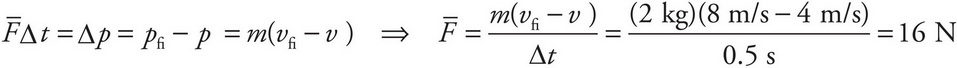# AP Physics 1 Question 222: Answer and Explanation

### Test Information

Question: 222

3. A box with a mass of 2 kg accelerates in a straight line from 4 m/s to 8 m/s due to the application of a force whose duration is 0.5 s. Find the average strength of this force.

• A. 4 N
• B. 8 N
• C. 12 N
• D. 16 N